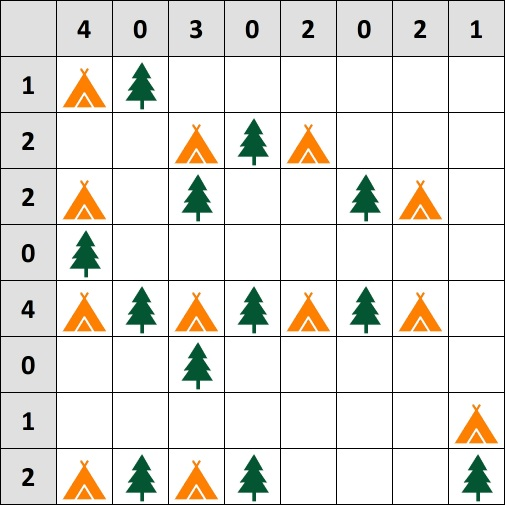# Tents

A single player game in which trees are arranged in a grid and the player places pairwise non-adjacent tents next to them so that trees and tents can be matched.## Description

Tents is a single player game played on a rectangular grid. Initially, each cell of the grid is either empty or it is occupied by a tree. A hint, i.e., a non-negative integer, is associated with each row and each column of the grid.

The player can place tents into empty cells with the goal of satisfying the following conditions:

• Each cell contains at most one tent.
• No two horizontally, vertically, or diagonally adjacent cells both contain a tent.
• The number of tents in each row (resp. column) is equal to the hint associated with the corresponding row (resp. column).
• There is a perfect matching between trees and tents so that each matched tree is matched to an orthogonally adjacent tree.

The figure shows a solved instance of Tents.

## Computational complexity

The problem of deciding whether an instance of Tents admits a solution is NP-Complete .

The variant of the problem in which there are no hints (and hence the number of tents in each row/column is unconstrained) is also NP-Complete .

Picture by Wikipedia user Tulp8 (CC BY-SA 4.0).M. De Biasi, “The Complexity of Camping”.

@misc{cog:tents,
author = "{CoG contributors}",
title  = "{Tents --- Complexity of Games}",
year   = "2022",
url    = "https://www.isnphard.com/i/tents/",
note   = "[Online; accessed 2022-06-02]"
}